Question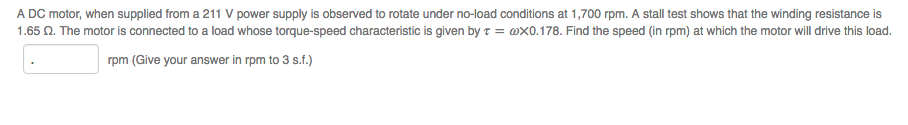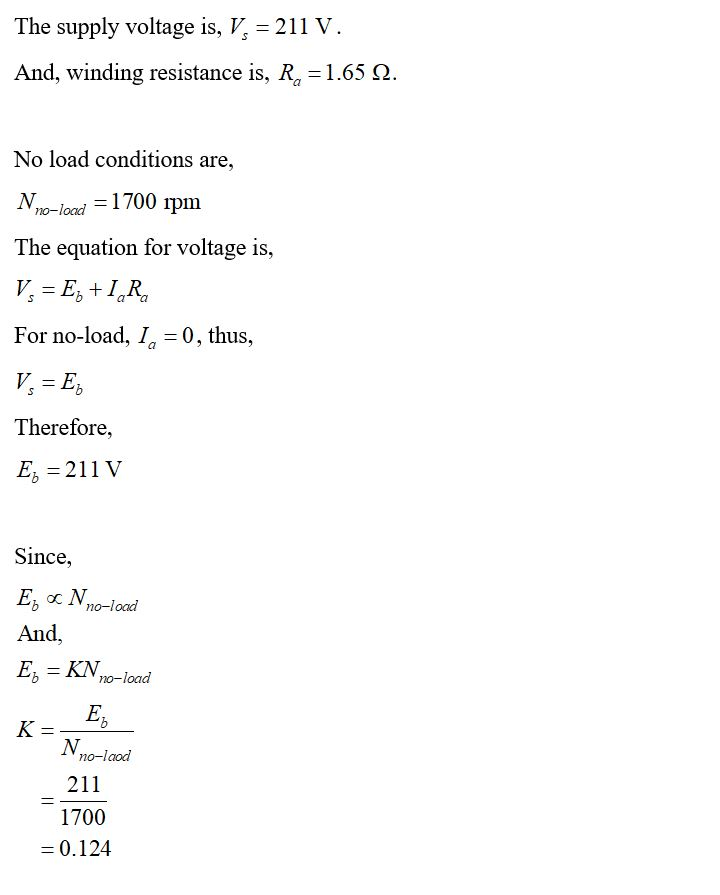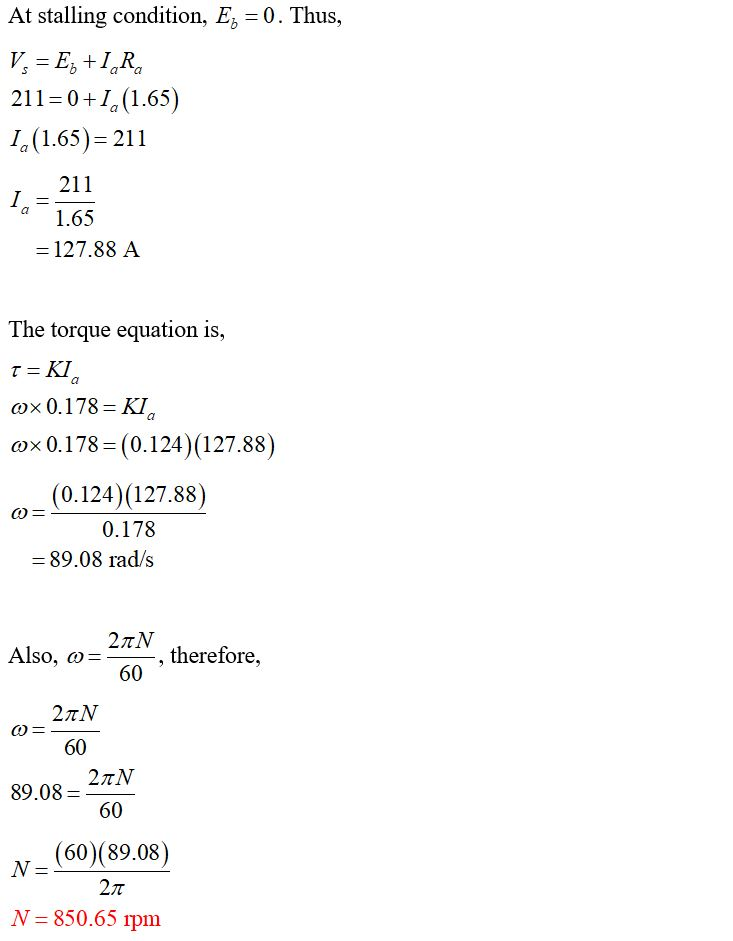#### Earn Coins

Coins can be redeemed for fabulous gifts.

Similar Homework Help Questions
• ### A DC motor, when supplied from a 90 V power supply is observed to rotate under...

A DC motor, when supplied from a 90 V power supply is observed to rotate under no-load conditions at 1,200 rpm. A stall test shows that the winding resistance is 2 Ω. Assume a linear model for the DC machine as presented in lectures. What is the back-emf constant for this machine? Give your answer in V.s.rad-1 to 3 decimal places.

• ### Q1b) DC Machines [ 6 marks ] You are given a 24 V DC motor. When...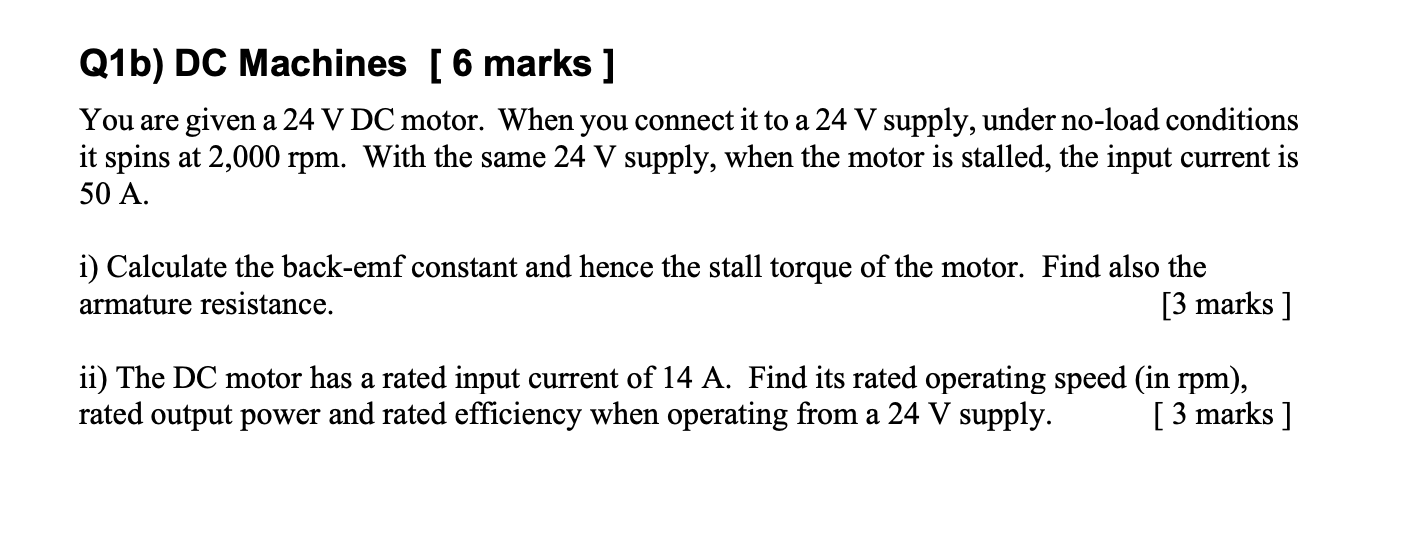Q1b) DC Machines [ 6 marks ] You are given a 24 V DC motor. When you connect it to a 24 V supply, under no-load conditions it spins at 2,000 rpm. With the same 24 V supply, when the motor is stalled, the input current is 50 A. i) Calculate the back-emf constant and hence the stall torque of the motor. Find also the armature resistance. [3 marks ] ii) The DC motor has a rated input current of...

• ### need the answer for the question no. 1 ENGR 113 Homework DC Motor Objectives Plot the...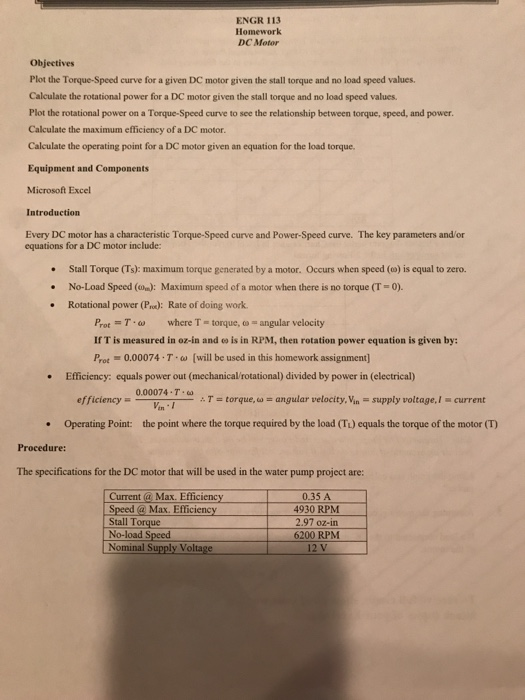need the answer for the question no. 1 ENGR 113 Homework DC Motor Objectives Plot the Torque-Speed curve for a given DC motor given the stall torque and no load speed values Calculate the rotational power for a DC motor given the stall torque and no load speed values Plot the rotational power on a Torque-Speed curve to see the relationship between torque, speed, and power Calculate the maximum efficiency of a DC motor. Calculate the operating point for a...

• ### A 6 hp, 180 V shunt dc motor draws a full-load current of 30 A from the power supply. The motor h...

A 6 hp, 180 V shunt dc motor draws a full-load current of 30 A from the power supply. The motor has a field (shunt) resistance of 150 Ω. The armature resistance of the motor is 0.1 Ω. When the motor speed is 1800 rpm (this is not the full-load speed), the relationship between the induced voltage

• ### Question 3: DC motors can be simplistically modeled as shown in Figure 5 MoTor back emf Vi: +1 Re...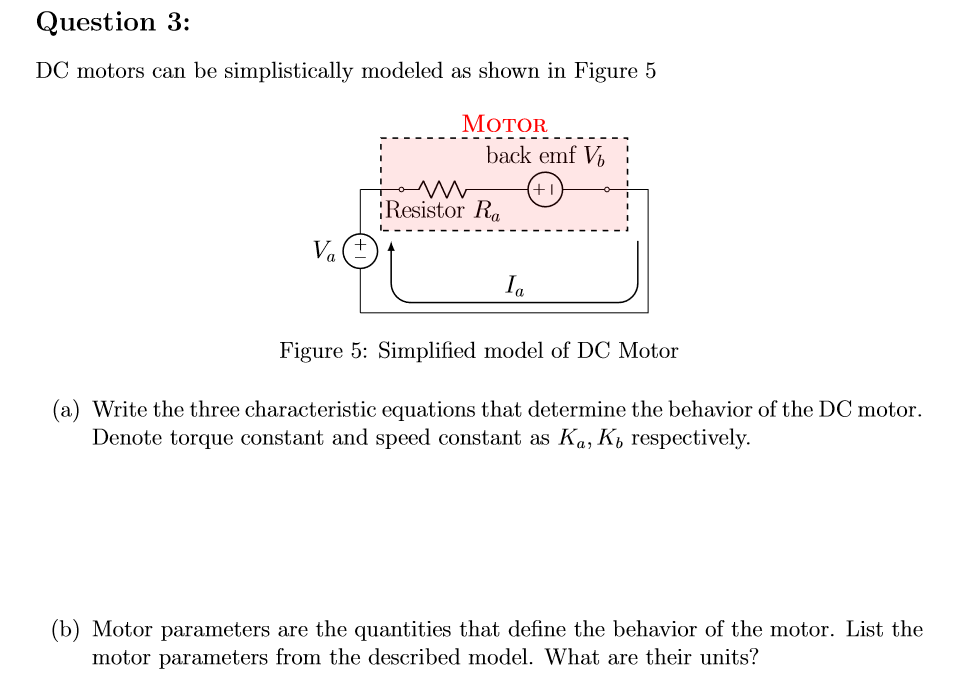Question 3: DC motors can be simplistically modeled as shown in Figure 5 MoTor back emf Vi: +1 Resistor Ra Ia Figure 5: Simplified model of DC Motor (a) Write the three characteristic equations that determine the behavior of the DC motor. Denote torque constant and speed constant as Ka, K, respectively. (b) Motor parameters are the quantities that define the behavior of the motor. List the motor parameters from the described model. What are their units? (c) For given...

• ### For a PMDC motor powered by a 12 V DC source, the no-load speed and stall...

For a PMDC motor powered by a 12 V DC source, the no-load speed and stall torque are 3800 RPM and 30 mNm, respectively. If the motor is running at 2500 RPM, find a) Shaft torque (Tshaft), b) Rotor current (Im), c) Back emf (Eb), d) Electrical power consumed, e) Shaft power, and f) Power loss in rotor coil.

• ### If a manufacturer's specifications for a PM DC motor are as follows, what are the motor's...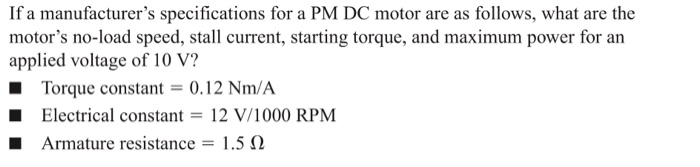If a manufacturer's specifications for a PM DC motor are as follows, what are the motor's no-load speed, stall current, starting torque, and maximum power for an applied voltage of 10 V? ■ Torque constant 0.12 Nm/A Electrical constant 12 V/1000 RPM Armature resistance-: 1.5 Ω

• ### A 250-V shunt-connected DC motor runs at 1500 rpm when it is not connected to any...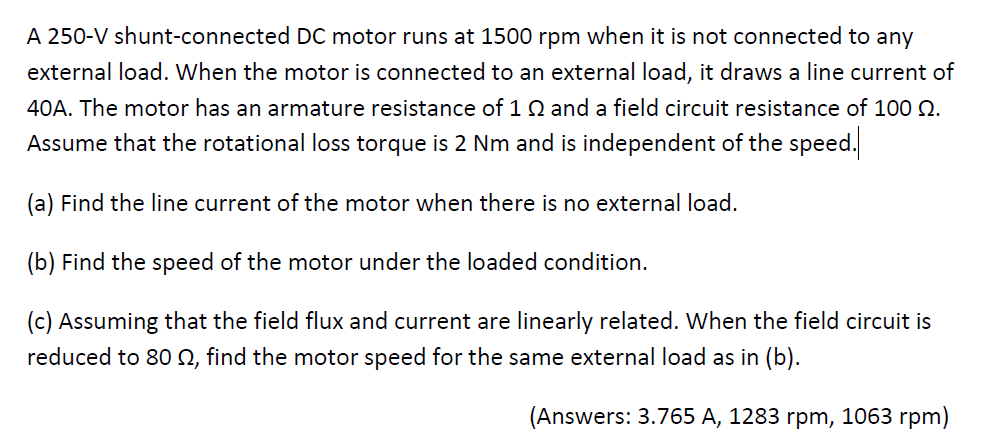A 250-V shunt-connected DC motor runs at 1500 rpm when it is not connected to any external load. When the motor is connected to an external load, it draws a line current of 40A. The motor has an armature resistance of 1 ? and a field circuit resistance of 100 ?. Assume that the rotational loss torque is 2 Nm and is independent of the speed (a) Find the line current of the motor when there is no external load....

• ### The permanent DC motor model CDP3450 by ABB is rated at 1 Hp, 2 500 RMP...

The permanent DC motor model CDP3450 by ABB is rated at 1 Hp, 2 500 RMP at 90v (no load). The armature resistance is 0.425 Ohm. a. The continuous current rating of this motor is 9.7 Amp, what is the maximum continuous torque rating? b. The motor is to drive a load of 2NM torque while maintaining a constant speed of 2000 RPM. What is the required supply current and voltage? c. With 90v armature voltage, what is the maximum...

• ### A DC motor supplied from 12 V draws 1.25 amps and turns at 1200 RPM when...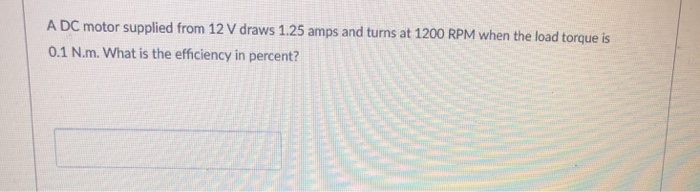A DC motor supplied from 12 V draws 1.25 amps and turns at 1200 RPM when the load torque is 0.1 N.m. What is the efficiency in percent?